## Tuesday, December 6, 2022

### Adjustable color palette for the Mk model plotting function, `plot.Qmatrix`

Yesterday I posted about how the default color palette of `phytools::contMap` should probably not be used – it is not colorblind friendly & renders poorly if converted to a grey color scale.

This is easy to adjust using the phytools function `setMap` (which also works for other `"contMap"` like graphs, such as the plotting method for `multirateBM`).

However, there are other plotting functions in phytools whose colors are not as easy to adjust. I just pushed a small update to one of these: `plot.Qmatrix`. This is the S3 `plot` method used for Q matrices (the object of class `"Qmatrix"`) as well as fitted model objects from `phytools::fitMk` and `geiger::fitDiscrete`.

Let’s see how this works.

First, I’m going to load phytools and an example dataset used in my recent book with Luke Harmon.

``````## load phytools
library(phytools)
## check package version number
packageVersion("phytools")
``````
``````##  '1.3.0'
``````
``````## load tree & data
row.names=1)
ecomorph.tree<-drop.tip(anole.tree,
geiger::name.check(anole.tree,anole.ecomorphs)\$tree_not_data)
ecomorph<-setNames(anole.ecomorphs\$ecomorph,row.names(anole.ecomorphs))
``````

Now I’ll fit the “all-rates-different” (`"ARD"`) model to these data. I’m not arguing this is a particularly good model; however, it is one that gives us lots of different rates to plot!

``````## fit ARD model
fitARD<-fitMk(ecomorph.tree,ecomorph,model="ARD",pi="fitzjohn",
opt.method="optimParallel")
fitARD
``````
``````## Object of class "fitMk".
##
## Fitted (or set) value of Q:
##           CG       GB        TC        TG       Tr        Tw
## CG -0.000002 0.000000  0.000000  0.000000 0.000000  0.000001
## GB  0.000000 0.000000  0.000000  0.000000 0.000000  0.000000
## TC  0.379452 0.000000 -0.594337  0.000000 0.214884  0.000000
## TG  0.090593 0.334218  0.084092 -1.002463 0.072221  0.421338
## Tr  0.000000 0.000000  0.000000  0.000000 0.000000  0.000000
## Tw  0.000000 0.330015  0.768605  0.000000 0.000001 -1.098622
##
## Fitted (or set) value of pi:
## CG GB TC TG Tr Tw
##  0  0  0  1  0  0
## due to treating the root prior as (a) nuisance.
##
## Log-likelihood: -68.387541
##
## Optimization method used was "optimParallel"
``````

Now, let’s plot it using the default color palette in `plot.Qmatrix`.

``````plot(fitARD,color=TRUE,lwd=3,tol=1e-2)
``````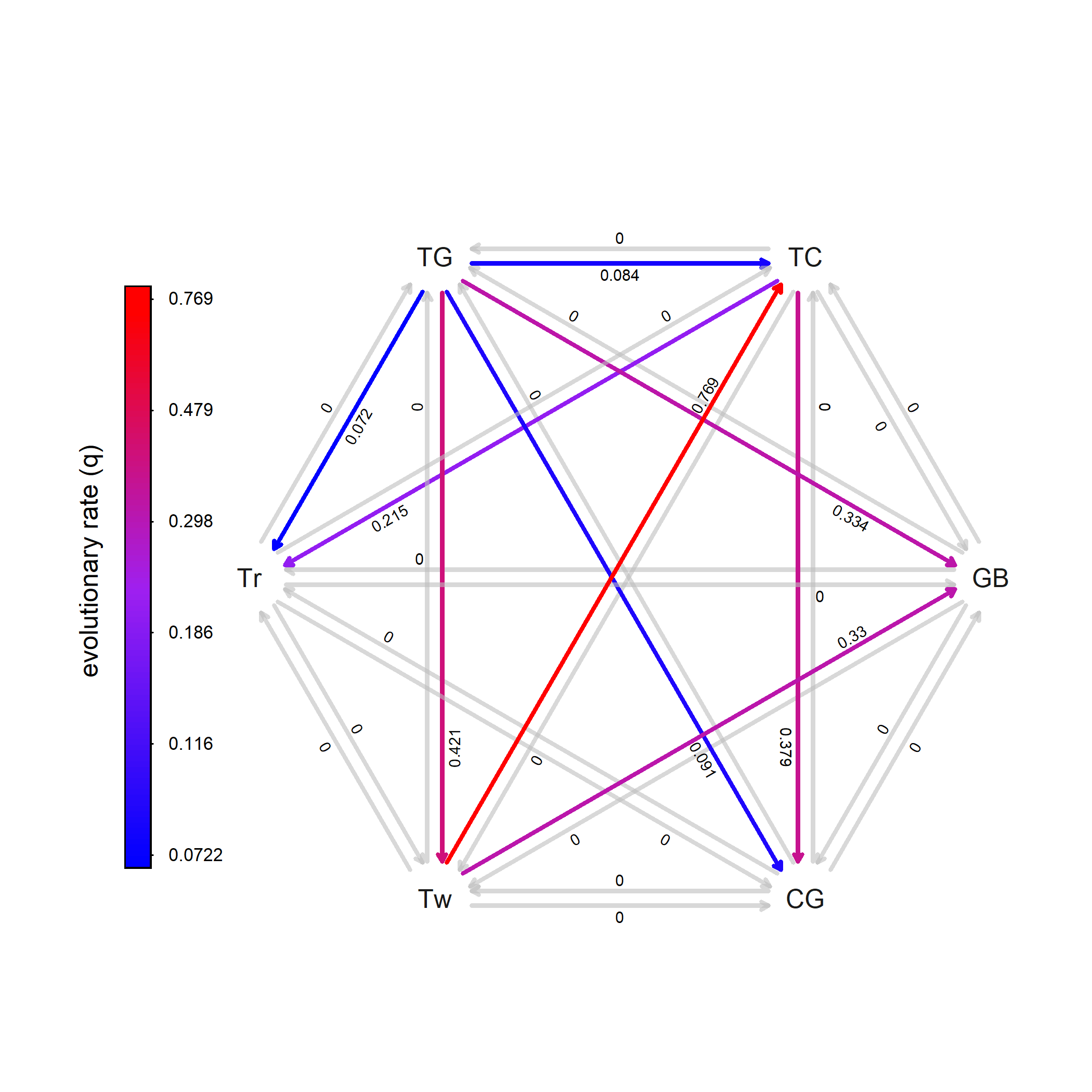To simulate how this would look when rendered into grey scale, we need to use `TeachingDemos::col2grey` again, but this time with the new `plot.Qmatrix` argument `palette` as follows.

``````cols<-colorRampPalette(c("blue", "purple", "red"))(20)
plot(fitARD,color=TRUE,lwd=3,tol=1e-2,
palette=TeachingDemos::col2grey(cols))
``````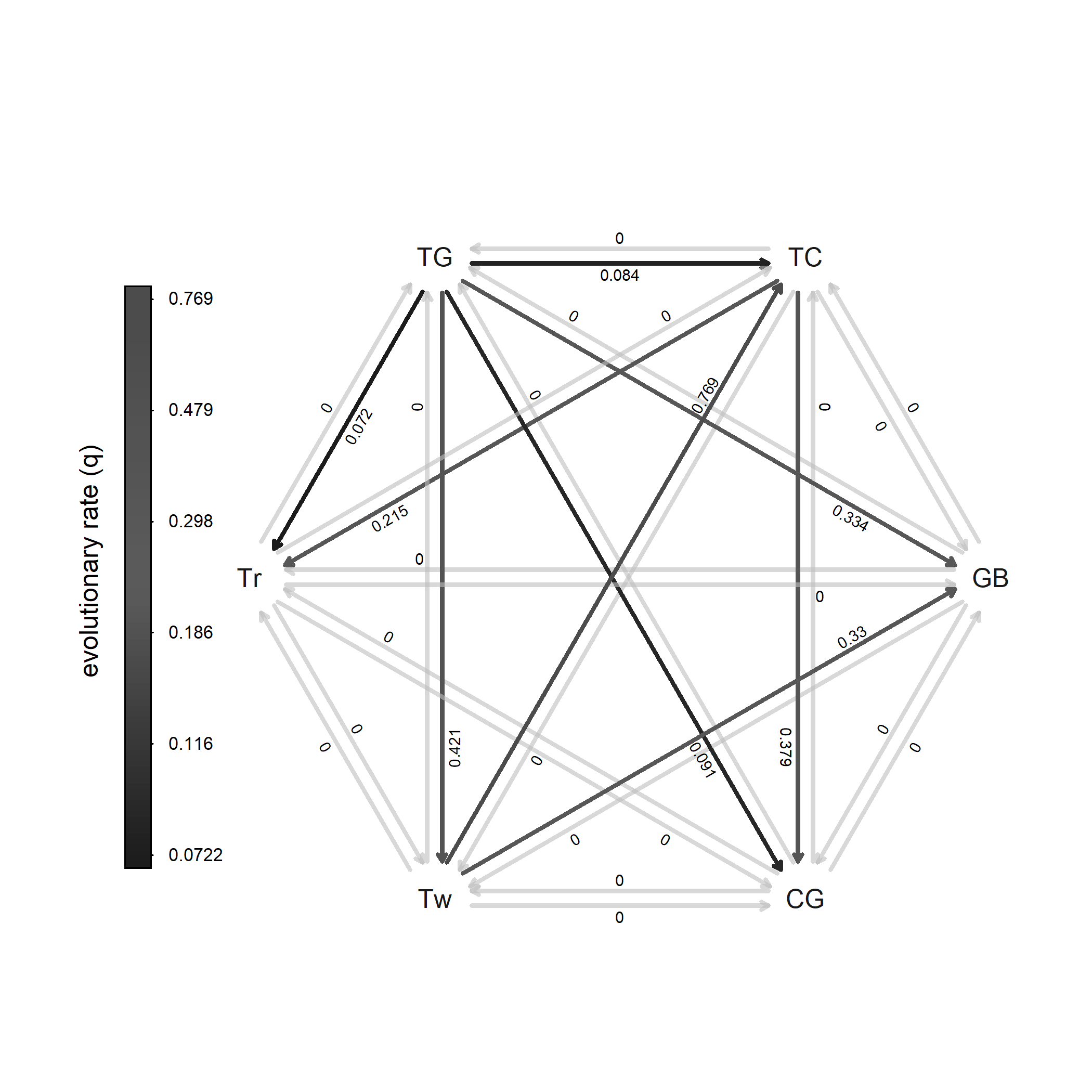Obviously, that’s no good!

As before, let’s try the ‘viridis’ palette using `viridisLite::viridis`. First in color:

``````cols<-viridisLite::viridis(n=20)
plot(fitARD,color=TRUE,lwd=3,palette=cols,tol=1e-2)
``````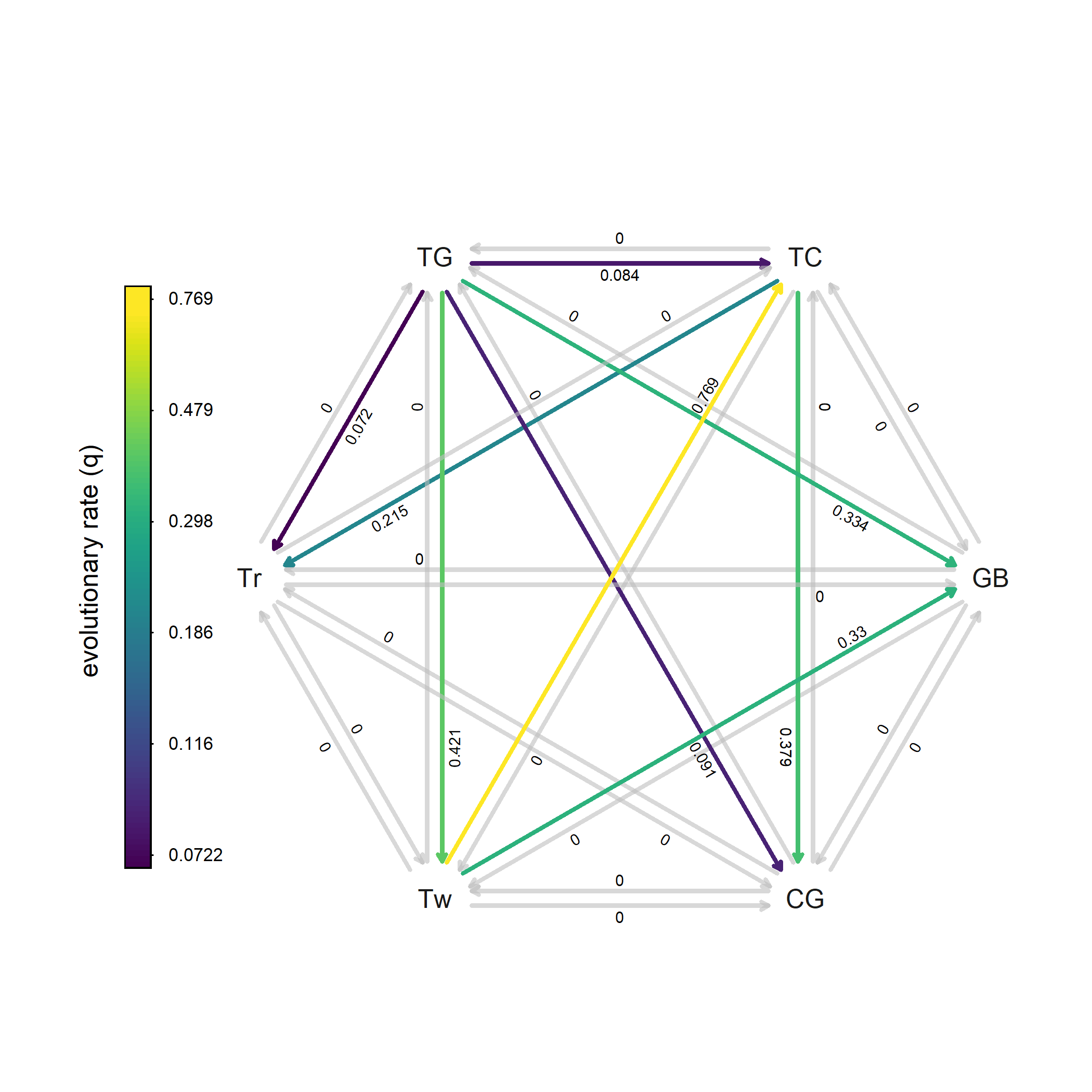Now, rendered into grey scale:

``````plot(fitARD,color=TRUE,lwd=3,tol=1e-2,
palette=TeachingDemos::col2grey(cols))
``````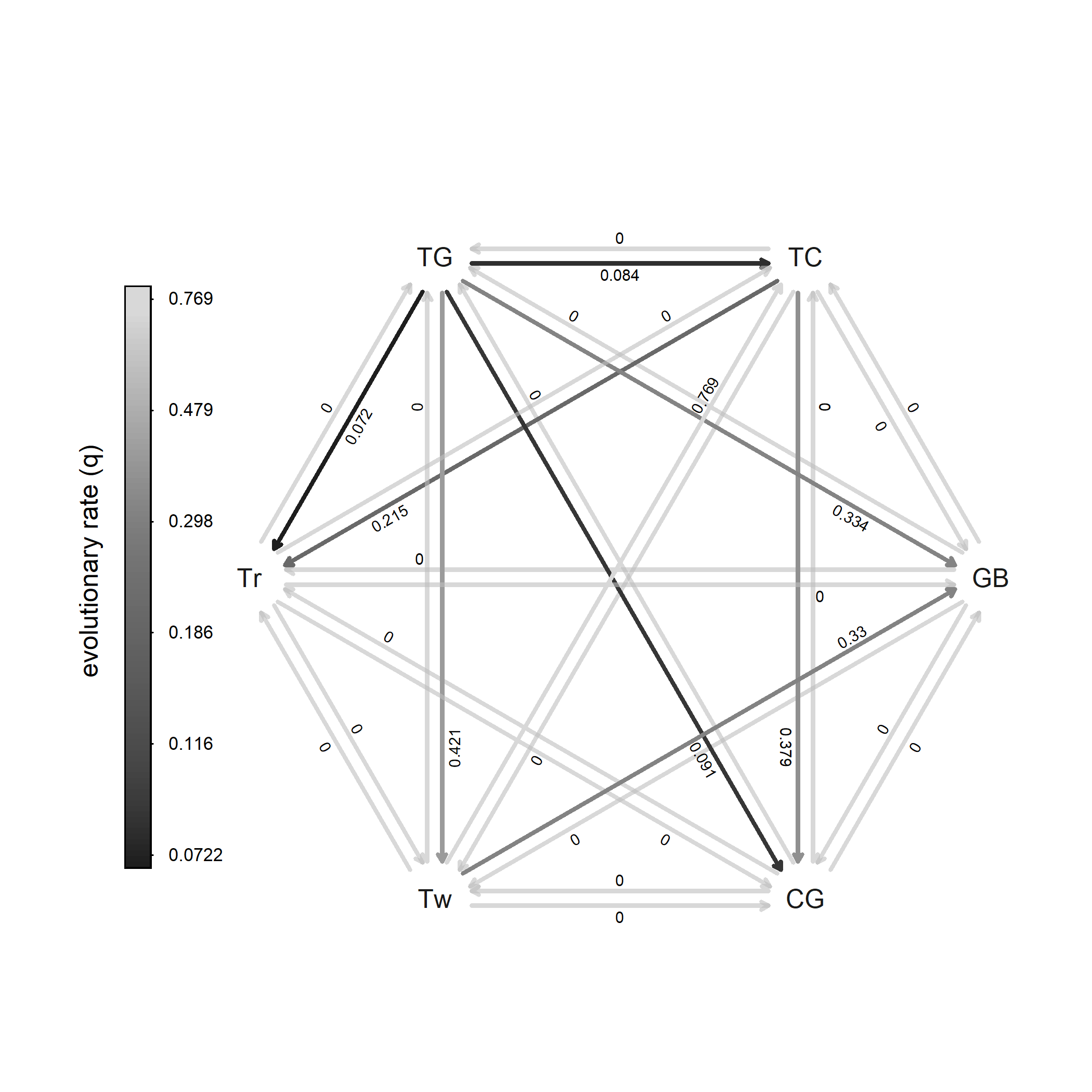Although this is obviously much better, something that we see right away is that our zero rates (which are shown using a grey color) are indistinguishable from our high rates. We can fix this either by using `show.zeros=FALSE`, e.g.:

``````plot(fitARD,color=TRUE,lwd=3,tol=1e-2,
palette=TeachingDemos::col2grey(cols),
show.zeros=FALSE)
``````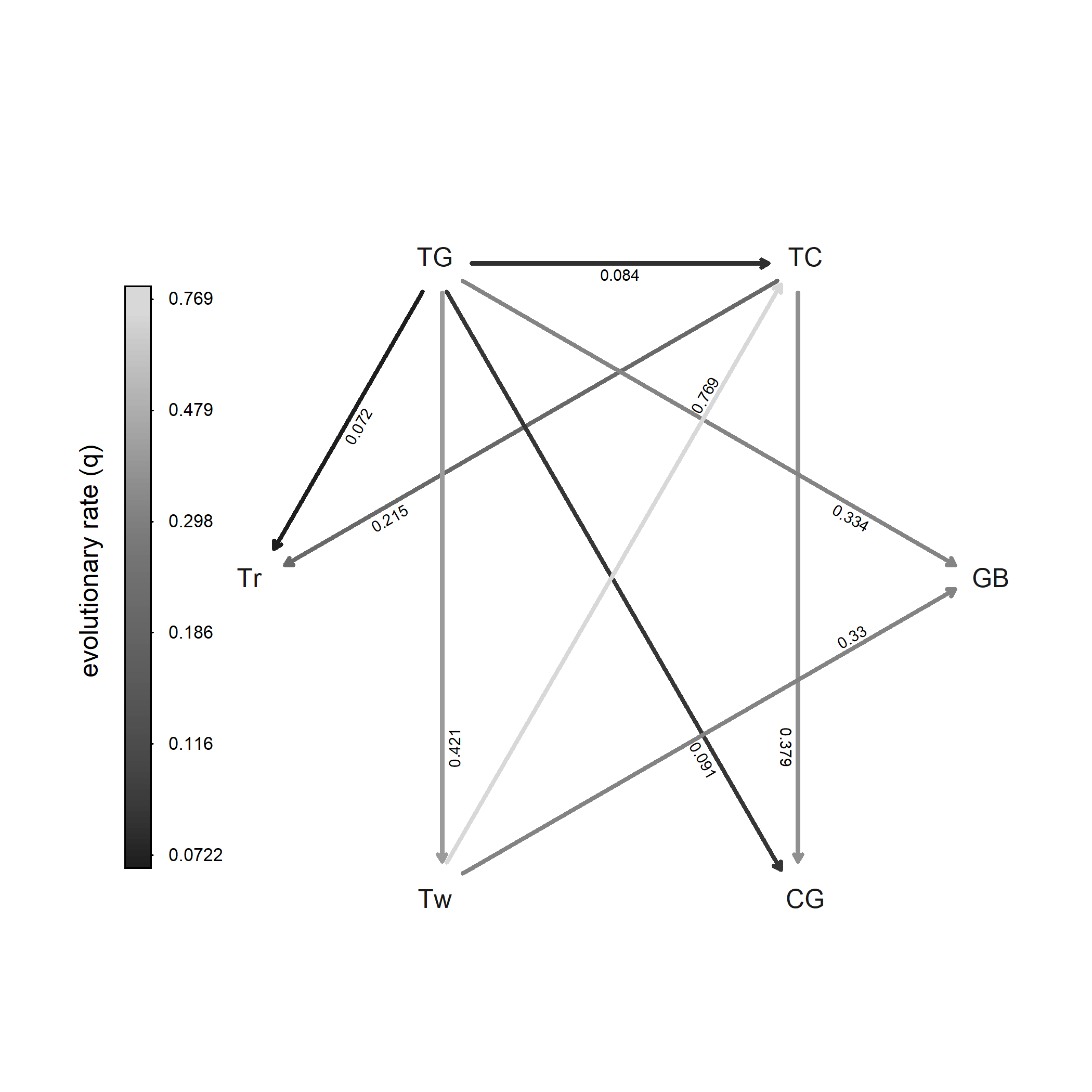Or, perhaps even better, by turning on `width=TRUE`.

``````plot(fitARD,color=TRUE,lwd=3,tol=1e-2,
palette=TeachingDemos::col2grey(cols),
width=TRUE)
``````